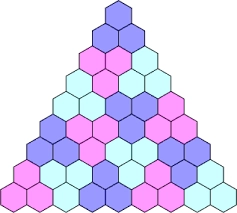# Difference 81849

Determine four numbers so that the first three form the successive three terms of an arithmetic sequence with difference d=-3 and the last three form the next terms of a geometric sequence with quotient q=one half.

a1 =  9
a2 =  6
a3 =  3
a4 =  1.5

### Step-by-step explanation:Did you find an error or inaccuracy? Feel free to write us. Thank you!

Tips for related online calculators
Do you have a linear equation or system of equations and looking for its solution? Or do you have a quadratic equation?